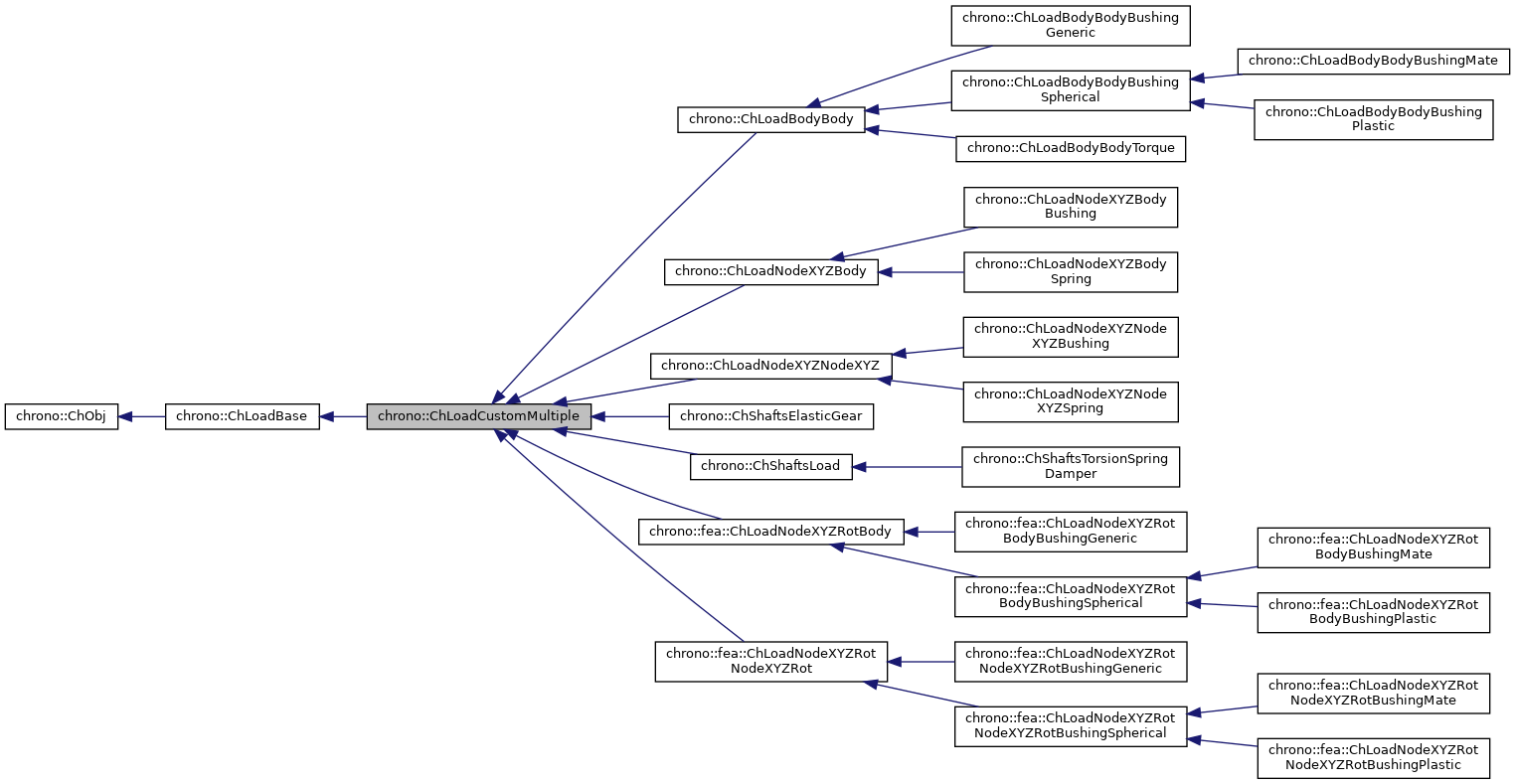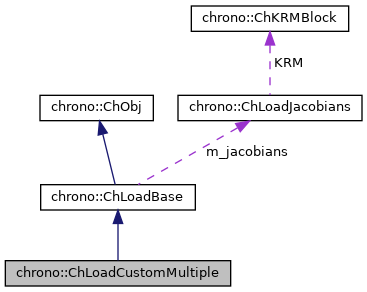## Description

One must inherit from this and implement ComputeQ() directly. The ComputeQ() must write the generalized forces Q into the "load_Q" vector of this object. Given that multiple ChLoadable objects are referenced here, their sub-forces Q are assumed appended in sequence in the "load_Q" vector, in the same order that has been used in the std::vector "mloadables" for ChLoadCustomMultiple creation. The same applies for the order of the sub-matrices of jacobians K,R etc.

#include <ChLoad.h>[legend][legend]

## Public Member Functions

Gets the number of DOFs affected by this load (position part)

Gets the number of DOFs affected by this load (speed part)

virtual void LoadGetStateBlock_x (ChState &mD) override
Gets all the current DOFs packed in a single vector (position part)

virtual void LoadGetStateBlock_w (ChStateDelta &mD) override
Gets all the current DOFs packed in a single vector (speed part)

virtual void LoadStateIncrement (const ChState &x, const ChStateDelta &dw, ChState &x_new) override
Increment a packed state (ex. More...

Number of coordinates in the interpolated field, ex=3 for a tetrahedron finite element or a cable, = 1 for a thermal problem, etc.

virtual void ComputeJacobian (ChState *state_x, ChStateDelta *state_w, ChMatrixRef mK, ChMatrixRef mR, ChMatrixRef mM) override
Compute jacobians (default fallback). More...

Adds the internal loads Q (pasted at global nodes offsets) into a global vector R, multiplied by a scaling factor c, as R += forces * c.

virtual void LoadIntLoadResidual_Mv (ChVectorDynamic<> &R, const ChVectorDynamic<> &w, const double c) override
Default fallback: compute jacobians via ComputeJacobian(), then use M=-dQ/da to do R += c*M*w. More...

virtual void CreateJacobianMatrices () override
Create the jacobian loads if needed, and also set the ChVariables referenced by the sparse KRM block.

virtual ChVectorDynamicGetQ ()
Access the generalized load vector Q.Public Member Functions inherited from chrono::ChLoadBase
virtual void ComputeQ (ChState *state_x, ChStateDelta *state_w)=0
Compute Q, the generalized load(s). More...

Access the jacobians (if any, i.e. if this is a stiff load)

virtual void Update (double time)
Update: this is called at least at each time step. More...

virtual bool IsStiff ()=0
Report if this is load is stiff. More...

virtual void InjectKRMmatrices (ChSystemDescriptor &mdescriptor)
Tell to a system descriptor that there are item(s) of type ChKblock in this object (for further passing it to a solver)

virtual void KRMmatricesLoad (double Kfactor, double Rfactor, double Mfactor)
Adds the current stiffness K and damping R and mass M matrices in encapsulated ChKblock item(s), if any. More...Public Member Functions inherited from chrono::ChObj
ChObj (const ChObj &other)

virtual ChObjClone () const =0
"Virtual" copy constructor. More...

int GetIdentifier () const
Gets the numerical identifier of the object.

void SetIdentifier (int id)
Sets the numerical identifier of the object.

double GetChTime () const
Gets the simulation time of this object.

void SetChTime (double m_time)
Sets the simulation time of this object.

const char * GetName () const
Gets the name of the object as C Ascii null-terminated string -for reading only!

void SetName (const char myname[])
Sets the name of this object, as ascii string.

std::string GetNameString () const
Gets the name of the object as C Ascii null-terminated string.

void SetNameString (const std::string &myname)
Sets the name of this object, as std::string.

void MFlagsSetAllOFF (int &mflag)

void MFlagsSetAllON (int &mflag)

void MFlagSetON (int &mflag, int mask)

void MFlagSetOFF (int &mflag, int mask)

int MFlagGet (int &mflag, int mask)

virtual void ArchiveOUT (ChArchiveOut &marchive)
Method to allow serialization of transient data to archives.

virtual void ArchiveIN (ChArchiveIn &marchive)
Method to allow de-serialization of transient data from archives.

virtual std::string & ArchiveContainerName ()

## Public AttributesProtected Attributes inherited from chrono::ChLoadBaseProtected Attributes inherited from chrono::ChObj
double ChTime
the time of simulation for the object

## ◆ ComputeJacobian()

 void chrono::ChLoadCustomMultiple::ComputeJacobian ( ChState * state_x, ChStateDelta * state_w, ChMatrixRef mK, ChMatrixRef mR, ChMatrixRef mM )
overridevirtual

Compute jacobians (default fallback).

Compute the K=-dQ/dx, R=-dQ/dv , M=-dQ/da jacobians. Uses a numerical differentiation for computing K, R, M jacobians, if stiff load. If possible, override this with an analytical jacobian. NOTE: Given that multiple ChLoadable objects are referenced here, sub-matrices of mK,mR are assumed pasted in i,j block-positions where i,j reflect the same order that has been used in the std::vector "mloadables" at ChLoadCustomMultiple creation. Called automatically at each Update().

Parameters
 state_x state position to evaluate jacobians state_w state speed to evaluate jacobians mK result -dQ/dx mR result -dQ/dv mM result -dQ/da

overridevirtual

Default fallback: compute jacobians via ComputeJacobian(), then use M=-dQ/da to do R += c*M*w.

If possible, override this to bypass jacobian computation, if analytical expression c*M*w is known. Not needed (ex. override to {} ) if no M is involved, ex. no inertial effects. a scaling factor

Parameters
 R result: the R residual, R += c*M*w w the w vector

 void chrono::ChLoadCustomMultiple::LoadStateIncrement ( const ChState & x, const ChStateDelta & dw, ChState & x_new )
overridevirtual

Increment a packed state (ex.

as obtained by LoadGetStateBlock_x()) by a given packed state-delta. Compute: x_new = x + dw. Ex. this is called by the BDF numerical differentiation routine that computes jacobian in the default ComputeJacobian() fallback, if not overriding ComputeJacobian() with an analytical form.